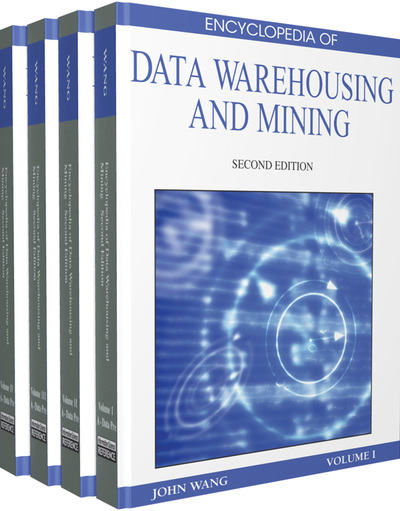# Flexible Mining of Association Rules

Hong Shen (Japan Advanced Institute of Science and Technology, Japan)
DOI: 10.4018/978-1-60566-010-3.ch137
Available
\$37.50
No Current Special Offers

## Abstract

The discovery of association rules showing conditions of data co-occurrence has attracted the most attention in data mining. An example of an association rule is the rule “the customer who bought bread and butter also bought milk,” expressed by T(bread; butter)? T(milk). Let I ={x1,x2,…,xm} be a set of (data) items, called the domain; let D be a collection of records (transactions), where each record, T, has a unique identifier and contains a subset of items in I. We define itemset to be a set of items drawn from I and denote an itemset containing k items to be k-itemset. The support of itemset X, denoted by Ã(X/D), is the ratio of the number of records (in D) containing X to the total number of records in D. An association rule is an implication rule ?Y, where X; ? I and X ?Y=0. The confidence of ? Y is the ratio of s(?Y/D) to s(X/D), indicating that the percentage of those containing X also contain Y. Based on the user-specified minimum support (minsup) and confidence (minconf), the following statements are true: An itemset X is frequent if s(X/D)> minsup, and an association rule ? XY is strong i ?XY is frequent and ( / ) ( / ) X Y D X Y ? ¸ minconf. The problem of mining association rules is to find all strong association rules, which can be divided into two subproblems: 1. Find all the frequent itemsets. 2. Generate all strong rules from all frequent itemsets. Because the second subproblem is relatively straightforward ? we can solve it by extracting every subset from an itemset and examining the ratio of its support; most of the previous studies (Agrawal, Imielinski, & Swami, 1993; Agrawal, Mannila, Srikant, Toivonen, & Verkamo, 1996; Park, Chen, & Yu, 1995; Savasere, Omiecinski, & Navathe, 1995) emphasized on developing efficient algorithms for the first subproblem. This article introduces two important techniques for association rule mining: (a) finding N most frequent itemsets and (b) mining multiple-level association rules.
Chapter Preview
Top

## Introduction

The discovery of association rules showing conditions of data co-occurrence has attracted the most attention in data mining. An example of an association rule is the rule “the customer who bought bread and butter also bought milk,” expressed by T(bread; butter)T(milk).

Let I ={x1,x2,…,xm} be a set of (data) items, called the domain; let D be a collection of records (transactions), where each record, T, has a unique identifier and contains a subset of items in I. We define itemset to be a set of items drawn from I and denote an itemset containing k items to be k-itemset. The support of itemset X, denoted by Ã(X/D), is the ratio of the number of records (in D) containing X to the total number of records in D. An association rule is an implication rule ⇒Y, where X; ⊆ I and X ΙY=0. The confidence of ⇒ Y is the ratio of σ(ΥY/D) to σ(X/D), indicating that the percentage of those containing X also contain Y. Based on the user-specified minimum support (minsup) and confidence (minconf), the following statements are true: An itemset X is frequent if σ(X/D)> minsup, and an association rule ⇒ XY is strong i ΥXY is frequent and ¸ minconf. The problem of mining association rules is to find all strong association rules, which can be divided into two subproblems:

• 1.

Find all the frequent itemsets.

• 2.

Generate all strong rules from all frequent itemsets.

Because the second subproblem is relatively straightforward — we can solve it by extracting every subset from an itemset and examining the ratio of its support; most of the previous studies (Agrawal, Imielinski, & Swami, 1993; Agrawal, Mannila, Srikant, Toivonen, & Verkamo, 1996; Park, Chen, & Yu, 1995; Savasere, Omiecinski, & Navathe, 1995) emphasized on developing efficient algorithms for the first subproblem.

This article introduces two important techniques for association rule mining: (a) finding N most frequent itemsets and (b) mining multiple-level association rules.

Top

## Background

An association rule is called binary association rule if all items (attributes) in the rule have only two values: 1 (yes) or 0 (no). Mining binary association rules was the first proposed data mining task and was studied most intensively. Centralized on the Apriori approach (Agrawal et al., 1993), various algorithms were proposed (Savasere et al., 1995; Shen, 1999; Shen, Liang, & Ng, 1999; Srikant & Agrawal, 1996). Almost all the algorithms observe the downward property that all the subsets of a frequent itemset must also be frequent, with different pruning strategies to reduce the search space. Apriori works by finding frequent k-itemsets from frequent (k-1)-itemsets iteratively for k=1, 2, , m-1.

Two alternative approaches, mining on domain partition (Shen, L., Shen, H., & Cheng, 1999) and mining based on knowledge network (Shen, 1999) were proposed. The first approach partitions items suitably into disjoint itemsets, and the second approach maps all records to individual items; both approaches aim to improve the bottleneck of Apriori that requires multiple phases of scans (read) on the database.

## Complete Chapter List

Search this Book:
Reset